Precalculus : Identify the Conic With a Given Polar Equation

Example Questions

← Previous 1

Example Question #11 : Conic Sections

Given the polar equation, determine the conic section: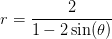Ellipse

Hyperbola

Parabola

Hyperbola

Explanation:

Recall that the polar equations of conic sections can come in the following forms: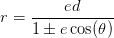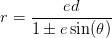, whereis the eccentricity of the conic section.

To determine what conic section the polar graph depicts, look only at the conic section's eccentricity.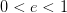will give an ellipse.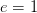will give a parabola.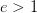will give a hyperbola.

Now, for the given conic section,so it must be a hyperbola.

Example Question #12 : Conic Sections

Given the polar equation, determine the conic section: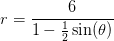Ellipse

Hyperbola

Parabola

Ellipse

Explanation:

Recall that the polar equations of conic sections can come in the following forms:, whereis the eccentricity of the conic section.

To determine what conic section the polar graph depicts, look only at the conic section's eccentricity.will give an ellipse.will give a parabola.will give a hyperbola.

Now, for the given conic section,so it must be an ellipse.

Example Question #1 : Polar Equations Of Conic Sections

Given the polar equation, determine the conic sectioN: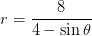Parabola

Hyperbola

Ellipse

Ellipse

Explanation:

Recall that the polar equations of conic sections can come in the following forms:, whereis the eccentricity of the conic section.

To determine what conic section the polar graph depicts, look only at the conic section's eccentricity.will give an ellipse.will give a parabola.will give a hyperbola.

First, put the given polar equation into one of the forms seen above by dividing everything by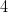.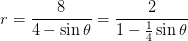Now, for the given conic section,so it must be an ellipse.

Example Question #22 : Conic Sections

Given the polar equation, determine the conic section: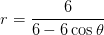Hyperbola

Parabola

Ellipse

Parabola

Explanation:

Recall that the polar equations of conic sections can come in the following forms:, whereis the eccentricity of the conic section.

To determine what conic section the polar graph depicts, look only at the conic section's eccentricity.will give an ellipse.will give a parabola.will give a hyperbola.

First, put the given polar equation into one of the forms seen above by dividing everything by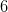.Now, for the given conic section,so it must be a parabola.

Example Question #1 : Polar Equations Of Conic Sections

Given the polar equation, determine the conic section: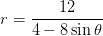Parabola

Ellipse

Hyperbola

Hyperbola

Explanation:

Recall that the polar equations of conic sections can come in the following forms:, whereis the eccentricity of the conic section.

To determine what conic section the polar graph depicts, look only at the conic section's eccentricity.will give an ellipse.will give a parabola.will give a hyperbola.

First, put the given polar equation into one of the forms seen above by dividing everything by.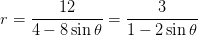Now, for the given conic section,so it must be a hyperbola.

Example Question #24 : Conic Sections

Given the polar equation, identify the conic section.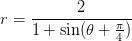Parabola

Hyperbola

Ellipse

Parabola

Explanation:

Recall that the polar equations of conic sections can come in the following forms:, whereis the eccentricity of the conic section.

To determine what conic section the polar graph depicts, look only at the conic section's eccentricity.will give an ellipse.will give a parabola.will give a hyperbola.

Now, for the given conic section,so it must be a parabola.

Example Question #25 : Conic Sections

Given the polar equation, identify the conic section: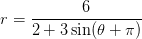Parabola

Hyperbola

Ellipse

Hyperbola

Explanation:

Recall that the polar equations of conic sections can come in the following forms:, whereis the eccentricity of the conic section.

To determine what conic section the polar graph depicts, look only at the conic section's eccentricity.will give an ellipse.will give a parabola.will give a hyperbola.

First, put the given polar equation into one of the forms seen above by dividing everything by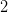.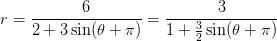Now, for the given conic section,so it must be a hyperbola.

Example Question #26 : Conic Sections

Given the polar equation, identify the conic section: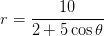Hyperbola

Ellipse

Parabola

Hyperbola

Explanation:

Recall that the polar equations of conic sections can come in the following forms:, whereis the eccentricity of the conic section.

To determine what conic section the polar graph depicts, look only at the conic section's eccentricity.will give an ellipse.will give a parabola.will give a hyperbola.

First, put the given polar equation into one of the forms seen above by dividing everything by.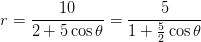Now, for the given conic section,so it must be a hyperbola.

Example Question #27 : Conic Sections

Given the polar equation, identify the conic section.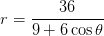Hyperbola

Ellipse

Parabola

Ellipse

Explanation:

Recall that the polar equations of conic sections can come in the following forms:, whereis the eccentricity of the conic section.

To determine what conic section the polar graph depicts, look only at the conic section's eccentricity.will give an ellipse.will give a parabola.will give a hyperbola.

First, put the given polar equation into one of the forms seen above by dividing everything by.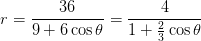Now, for the given conic section,so it must be an ellipse.

Example Question #28 : Conic Sections

Given the polar equation, identify the conic section.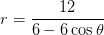Ellipse

Hyperbola

Parabola

Parabola

Explanation:

Recall that the polar equations of conic sections can come in the following forms:, whereis the eccentricity of the conic section.

To determine what conic section the polar graph depicts, look only at the conic section's eccentricity.will give an ellipse.will give a parabola.will give a hyperbola.

First, put the given polar equation into one of the forms seen above by dividing everything by.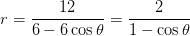Now, for the given conic section,so it must be a parabola.

← Previous 1

All Precalculus Resources